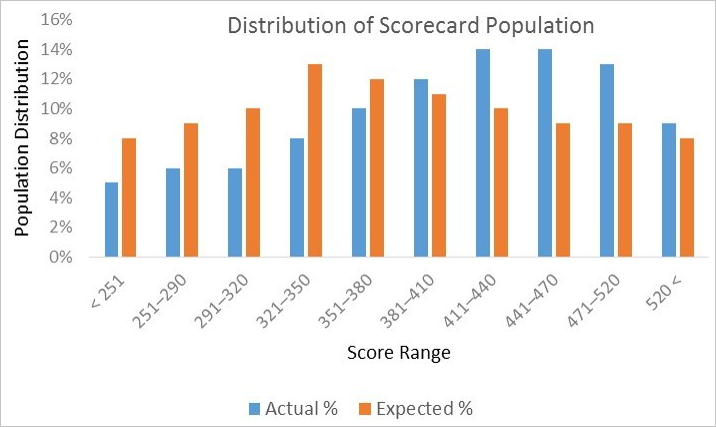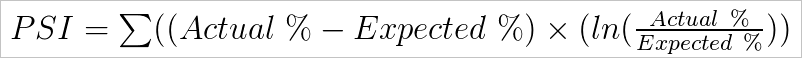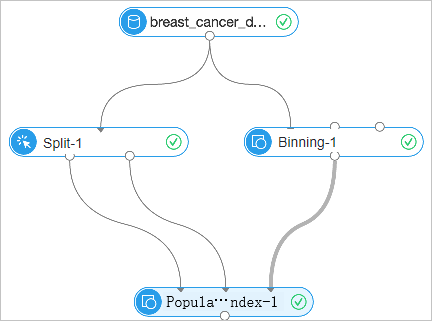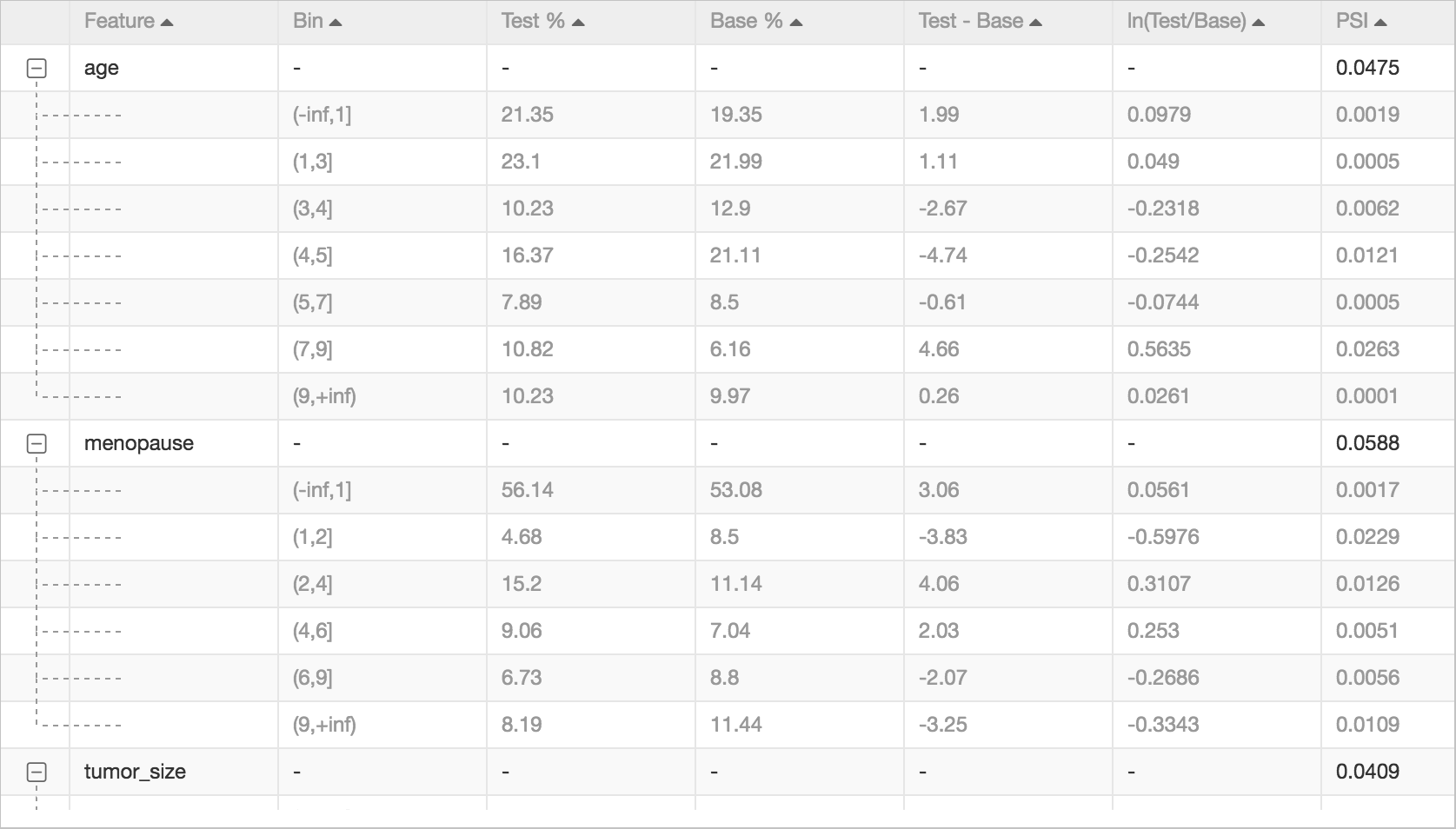Population stability index (PSI) is an important metric to identify a shift in two samples of a population.

## Background information

PSI is a common metric that is used to measure the stability of samples. For example, you can use it to measure whether the changes in the population within two months are stable. A PSI value less than 0.1 indicates insignificant changes. A PSI value from 0.1 to 0.25 indicates minor changes. A PSI value greater than 0.25 indicates major changes.

If the changes in a population over time are unstable, you can use charts to identify the changes. You can perform binning for variables, calculate the number and proportion of the samples in each bin, and present the statistics in a column chart. The following figure shows a sample chart.The preceding method can directly show whether a variable in two samples changes significantly. However, the shift in these changes cannot be measured by using this method. Therefore, the population stability cannot be automatically monitored. To resolve this issue, you can use the PSI component. The following figure shows the formula that is used to calculate PSI values.## Configure the component

You can configure the PSI component by using one of the following methods:
• Use the Machine Learning Platform for AI console
Tab Parameter Description
Fields Setting Features for PSI Calculation The feature columns that are required for PSI value calculation.
Tuning Number of Cores The number of CPU cores that are required. By default, the system determines the value.
Memory Size The memory size of each CPU core. By default, the system determines the value.
• Use commands
``````PAI -name psi
-project algo_public
-DinputBaseTableName=psi_base_table
-DinputTestTableName=psi_test_table
-DoutputTableName=psi_bin_table
-DinputBinTableName=pai_index_table
-DfeatureColNames=fea1,fea2,fea3
-Dlifecycle=7``````
Parameter Description Required Default value
inputBaseTableName The name of the base table. The shift of the population is calculated based on the samples in the base and test tables. Yes N/A
inputBaseTablePartitions The partitions that are selected from the base table. No Full table
inputTestTableName The name of the test table. The shift of the population is calculated based on the samples in the base and test tables. Yes N/A
inputTestTablePartitions The partitions that are selected from the test table. No Full table
inputBinTableName The name of the binning result table. Yes N/A
featureColNames The feature columns that are required for PSI value calculation. No Full table
outputTableName The name of the output table. Yes N/A
lifecycle The lifecycle of the output table. No N/A
coreNum The number of CPU cores that are required. No Determined by the system
memSizePerCore The memory size of each CPU core. Unit: MB. No Determined by the system

## Example

Use the Binning component to perform binning for features. Then, connect the PSI component to the two sample datasets that you want to compare and the Binning component, as shown in the following figure. Specify the Features for PSI Calculation parameter.The following figure shows the calculation results of the PSI component.